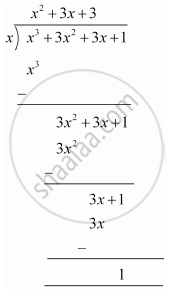# Find the remainder when x^3 + 3x^2 + 3x + 1 is divided by x. - Mathematics

Find the remainder when x3 + 3x2 + 3x + 1 is divided by x.

#### Solution 1

Let p(x) = x3 + 3x2 + 3x + 1

x = 0

∴ Remainder = (0)3 + 3(0)2 + 3(0) + 1

= 1

Therefore, the remainder is 1.

#### Solution 2

By long division,Therefore, the remainder is 1.

Concept: Remainder Theorem
Is there an error in this question or solution?

#### APPEARS IN

NCERT Class 9 Maths
Chapter 2 Polynomials
Exercise 2.3 | Q 1.3 | Page 40